## 2228 Reputation

17 years, 169 days

## Using cat...

I would suggest using

`cat("",i,".m")`

for the file names. Note that the first argument "" to cat is there for the sole purpose of making the concatenation have type 'string' as appropiate for a file name.

Update: I was thinking whether you know that you can save all your variables in a single file, for instance as a sequence of variables, see the help page on save; that is, whether your choice of saving one variable per file, which appears to me a bit wasteful, is fully deliberate.

## Using MakeUnique...

If using a list, instead of a set, is ok, then I might suggest the following:

```permUnique := (L::list) -> ListTools:-MakeUnique(L,1,(x,y) -> evalb(
x = eval(y,{a = b,b = c,c = a}) or
x = eval(y,{a = c,b = a,c = b})
)):
# Applied to the examples you give
permUnique([a,b,a^2,b^2,c,c + 2*a,a - b,c^2,a + 2*b,b + 2*c]);
permUnique([a*b,b*c,a - b^2,b - c^2,a^2,c - a^2]);```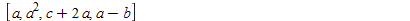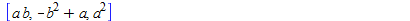Note by the way that you are missing * in various places.

## proc + module...

Personally, I prefer the following construction:

```createModule := proc(Input)
module()
option package;
export o1,o2,o3;
o1 := proc() ... end proc;
o2 := proc() ... end proc;
o3 := proc() ... end proc;
end module
end proc:```

because in this way Input figures only once. The dots indicate, of course, doing something using Input. It can then be used as follows:

```M := createModule(input):
M:-o1();
M:-o2();
M:-o3();```

there here being the advantage of being able, if desired, to pass further parameters, in excess of 'input' itself, to any of the functions o1,o2,o3.

## solve...

Perhaps I misunderstand you, but if you want a set of equations, eqs say, to be solved with respect to some of its indeterminates, inds say, then you can just write

`solve(eqs,inds);`

More generally, if you solve without specifying any indeterminates, i.e., just writes sol := solve(eqs), then the free parameters, if a solution exists, can be found using

`select(x -> lhs(x) = rhs(x),sol);`

And, perhaps more interestingly for you, the solution in terms of these free parameters can then be selected as follows:

`remove(x -> lhs(x) = rhs(x),sol);`

PS: In the above, I assume that the solution is unique, if it exists.

## Right...

I think you are right. For a moment, I thought that using Setup(g_[~mu,~nu] = some matrix); would solve the problem, but the following shows otherwise:

```g := Matrix([
[-1,0,0,2],
[ 0,1,0,0],
[ 0,0,1,0],
[ 2,0,0,1]
]):
Setup(g_[~mu,~nu] = g):
evalb(g_[~1,~1] = g[1,1]),
evalb(g_[ 1, 1] = g[1,1]);
g_[~1,~1],LinearAlgebra:-MatrixInverse(g)[1,1];```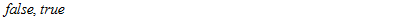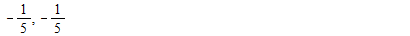It seems that Setup simply ignores the tilde signs. But, of course, there may possibly be other ways.

## Explicit sums...

As far as I can see, you have to perform explicit sums over the Pauli index a. This applies to your expression for U:

`U := 1+i*(1/f)*add(p[a](X)*Psigma[a],a = 1..3);`

and to your expression for the Trace over the squares of the Pauli matrices:

`Trace(add(Psigma[a]*Psigma[a],a = 1..3));`

PS: The command SumOverRepeatedIndices cannot be used here, because it is designed to sum over only spacetime indices. Maple 17, which I use, does not have the StandardModel package, including the possibility of setting up some su2 formalism, I guess, so these parts of your worksheet I cannot comment on.

## Substitution or Jet calculus...

Two approaches:

1.) Using substitution back and forth:

```expr := eval(L,diff(theta2(t),t) = u);
expr := diff(expr,u);
expr := eval(expr,u = diff(theta2(t),t));```

2.) Using the socalled Jet calculus (probably the most systematic/secure approach):

```expr := PDETools:-ToJet(L,theta2(t));
expr := diff(expr,theta2[t]);
expr := PDETools:-FromJet(expr,theta2(t));
expr := convert(expr,diff);```

Each approach can, of course, be generalized to include as well the time-derivative of theta3(t), if that is desired.

PS: Note that what you are doing is taking a functional derivative.

## A suggestion...

Perhaps the following is useful:

```L := zip((x,y) -> [x,y],A,B);
map(x -> `if`(x >= 0 and x <= 12,x,NULL),L)[-1];
map(x -> `if`(x > 12 and x <= 37,x,NULL),L)[-1];
map(x -> `if`(x >= 238             ,x,NULL),L)[-1];```

## Different entities...

Even though the output of diff(u(x, t), x, x) and u[x,x] look the same when PDETools is loaded and the declarations, using declare, have been performed, they are not the same:

```expr1 := diff(u(x,t),x,x);
expr2 := u[x,x];
op(expr1);
op(expr2);
T(expr1);
T(expr2);```

## Getting started...

Some preliminary considerations of mine:

1.) Setting up the metric:

```with(Physics):
Setup(mathematicalnotation = true):
Setup(coordinatesystem = cartesian):
Setup(metric = Matrix(4,4,(mu,nu) -> g_[mu,nu] + h[mu,nu](X),shape = symmetric)):
g_[];```

2.) The Christoffel symbols (of the second kind) that you want to calculate symbolically:

`convert(Christoffel[~rho,mu,nu],g_);`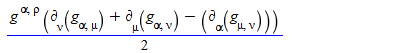Note that the expression you give is incorrect: the metric components should, as I am sure you know, not be covariantly differentiated (using semicolons), as that would of course make them vanish.

Now, the challenge is to expand these expressions to only lowest order in the components of h. Here a problem is that the command mtaylor cannot readily be applied because these entries are functions.

In Cartesian coordinates, where the derivatives of the metric are all purely first order in h, only the zero'th order of the inverse metric is needed. This being just -1, the calculations for that coordinate system are relatively straightforward. But, of course, for other coordinate systems, say spherical coordinates, no such simplification is readily available.

Update: The following auxiliary function can be used to linearize any expression with respect to the entries of h:

```linearize := proc(expr::algebraic)
local inds,sub1,sub2;
inds := indets(g_[]) minus {X,g_[mu,nu]};
inds := inds union `union`(seq(map(diff,inds,X[rho]),rho = 1..4));
sub1 := {seq(inds[i] = F||i,i = 1..nops(inds))};
sub2 := {seq(F||i = inds[i],i = 1..nops(inds))};
eval(mtaylor(eval(expand(expr),sub1),map(rhs,sub1),2),sub2);
end proc:```

Applied to the inverse metric, it yields:

`map(linearize,rhs(g_[~mu,~nu,matrix]));`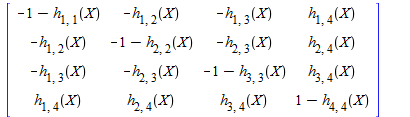More importantly, though, it may be applied to the components of the Christoffel connection:

`linearize(Christoffel[~1,2,3]);`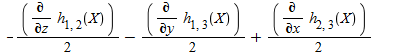## Eigenvectors...

I guess you can use the command Eigenvectors:

`M := LinearAlgebra:-Eigenvectors(A);`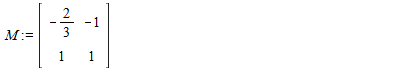## Keyword 'nonzero'...

The following example, using the Schwarzschild geometry, demonstrates how to extract nonzero components [from the Riemann tensor, as the Einstein tensor vanishes, of course, for that geometry]:

```with(Physics):
Setup(metric = sc):
Riemann[nonzero];
```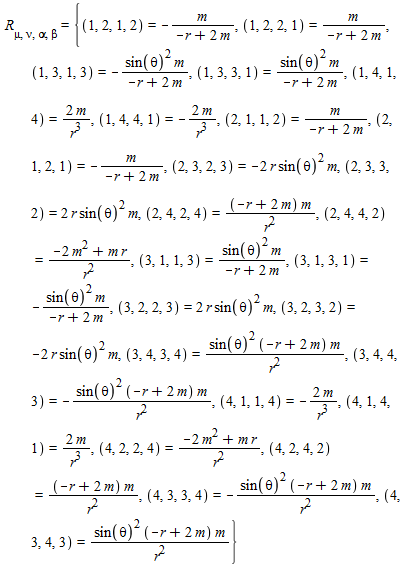Whether this format is readily acceptable for pdsolve, I have not considered; in fact, I am not sure what is being asked for.

## Coefficient matrix...

A suggestion:

```with(LinearAlgebra):
Matrix(Dimension(x),Dimension(u),(i,j) -> coeff(x[i],u[j]));```

## Squaring...

I guess that you are trying to set up the electroweak covariant derivative, right?

Breaking down the problem, the first issue I encounter is the squaring of the purely differential operator part of W, i.e., of Dgamma[~mu]*d_[mu]. It cannot just be done by 'naively' squaring it, as then Maple complains, rightfully so, that the index mu figures four times. The way to square it is as follows:

`Dgamma[~mu]*d_[mu]*Dgamma[~nu]*d_[nu];`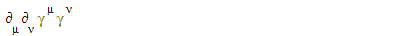from which

```SumOverRepeatedIndices(
Dgamma[~mu]*d_[mu]*Dgamma[~nu]*d_[nu]
);```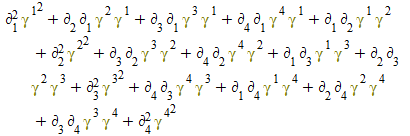This expression must then be simplified using the Clifford algebra for the Dirac gamma matrices. I have not yet figured out how to have Maple do that task. Taking the Trace:

```Trace(SumOverRepeatedIndices(
Dgamma[~mu]*d_[mu]*Dgamma[~nu]*d_[nu]
));```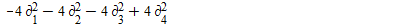does not count as a solution, as the correct result should of course be the product of the D'Alembertian and the 4x4 identity matrix. In that connection, I do not understand why Maple can evaluate Dgamma, say, but not Dgamma^2:

```eval(Dgamma),
eval(Dgamma^2);```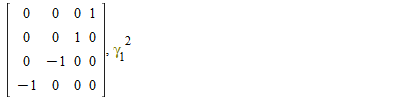PS: My version of the Physics package is 2014, March 19 (under Maple 17).

## Arguments as separate solutions...

If the two arguments of _F1 are separetely solutions to the differential equation, then of course any function of them, here _F1, will also be a solution to the differential equation [is that actually correct?]. And in fact they are, almost:

```pde := diff(rho(t,q,p),t)
+(diff(rho(t,q,p),q))*p
-(diff(rho(t,q,p),p))*(2*q+2):
sol := pdsolve(pde,rho(t,q,p)):
simplify(eval(pde,rho(t,q,p) = op(rhs(sol)))),
simplify(eval(pde,rho(t,q,p) = op(rhs(sol))));```

0, -csgn(p)+1

The second argument will be a solution only if p > 0, for only then does -csgn(p)+1 vanish. But why should only positive momenta be allowed? I guess the bug in pdsolve, noted above by Markiyan Hirnyk, is the culprit.

PS: I suppose your question is a continuation of the thread How do I solve a first order linear PDE with two time dependent variables?, which I have just revisited. I thought the thread was dead because it had no activity for two or three days. That is why I did not notice the latest comment of yours, posted, at the time of writing, 15 hours ago.

﻿